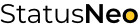WE ARE EXPERTS IN TECHNOLOGY

## Let’s Work Together# StatusNeo## Recursion in the Python Pandas DataFrames | Finding Hierarchy of Manager

#### Introduction

From the old book of Data structures, there are some concepts that sometimes help to solve the modern day professional problem. Recursion is one of them. By definition we know recursion is calling the same function by itself with a termination condition. It helps in solving the problem by calling a copy of itself to work on a smaller problem.

Each recursive call makes a new copy of that method (actually only the variables) in memory. Once a method ends, the copy of that returning method is removed from the memory. For better understand let take a dig into the visualization below:

#### Problem Statement

Find the hierarchy of the manager till the most junior employee. Manager ID will be passed in the argument of function. The data has 2 columns Employee ID and Manager ID.

Below screenshot displays the sample dataset considered for this problem.

#### Code and Explanation

So if we input ‘1’ manager ID as argument then the emp IDs in the result would be 2,3,4,5,6,13 ( reports directly to 1), then 7,8,9 (reports to 3) and 10,11,12 (reports to 2). So lets start by importing the Python libraries pandas and creating the pandas dataframe.

Now creating a function “levels” with argument as manager ID. Initializing the “childs” as empty dataframe with same columns as df. We have created global variable “childs” to remember the value from the previous calls of recursion.

Child_temp pandas dataframe is staging dataframe that will occupy the value of subset of df with respective values of employees matching with the manager ID (keeps on changing with subsequent call of levels function). Since, Pandas dataframe don’t support recursive query by default, so we have to use the loop within the recursive function to iterate between the rows. However, we are concatenating the empty “childs” dataframe with the staging one to get all the resultant records appended with each recursive call.

Here the values of childs_temp with each backtrace in recursion.

Once the if statement is not satisfied, else statement will terminate the calling the function itself. First rule of recursion, it should have base criteria to exit which will fulfill its backtrack process.

Thus, the all we need is to call the function in main.

#### Output and conclusion

Moreover, we get our desired output in df2 data frame.

In conclusion, that is just a logic, you can add it logically in any hierarchical problem of Pandas data frames. Recursive function is your child, you can do whatever you want. First rule of recursion, it should have base criteria to exit, it can have more than one criteria.